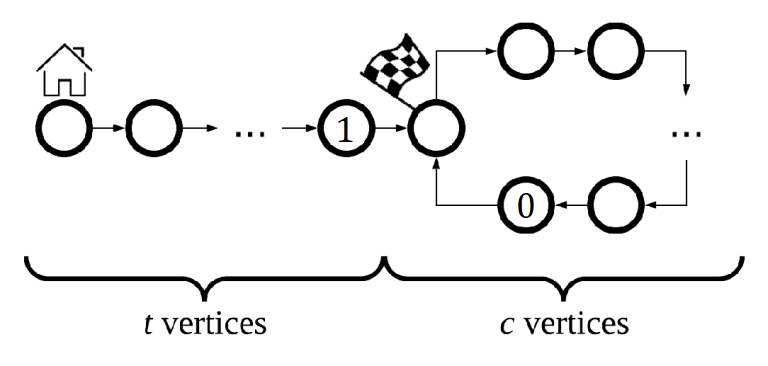2019-03-11 08:46:02

## 题解## 代码

#include<cstdio>
#include<cstring>
#include<vector>
//#define wjyyy
char s;
int main()
{
int sum=0,n,p=0;
while(++p)
{
printf("next 0 ");
if(!(p&1))
{
printf("1");
++sum;
}
puts("");
#ifndef wjyyy
fflush(stdout);
#endif
scanf("%d",&n);
int flag=0;
for(int i=1;i<=n;++i)
{
scanf("%s",s);
int tmp=0;
for(int j=0;s[j]!='\0';++j)
if(s[j]=='0'||s[j]=='1')
++tmp;
if(tmp==2)
flag=1;

}
if(flag)
break;
}
//环长是p-sum 1 走的是sum
bool g=(p-sum==sum);

while(n>1)
{
if(g)
puts("next 0 1 2 3 4 5 6 7 8 9");
else
puts("next 0 1");
g=1;
#ifndef wjyyy
fflush(stdout);
#endif
scanf("%d",&n);
int flag=0;
for(int i=1;i<=n;++i)
scanf("%s",s);
}
puts("done");
#ifndef wjyyy
fflush(stdout);
#endif
return 0;
}
• star
首页